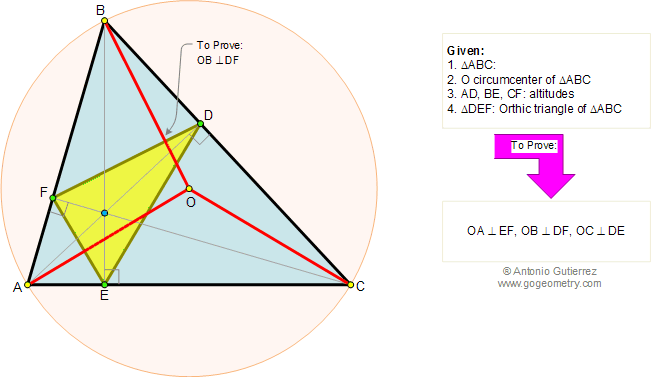# Geometry Problem 138. Nagel's Theorem, Orthic Triangle, Altitudes, Circumradius, Perpendicular.

In the figure below, given a triangle ABC of circumcenter O and its orthic triangle DEF (AD, BE, and CF are the altitudes of ABC). Prove that OA, OB, and OC are perpendicular to EF, DF, and DE respectively. View or post a solutionHome | Geometry | Altitude | Search | Problems | 131-140 | Orthic Triangle | Nagel | Email | View or post a solution